# BALANCING EQUATIONS NO 2 Balancing equations made easy

• Slides: 24BALANCING EQUATIONS NO 2 - Balancing equations made easy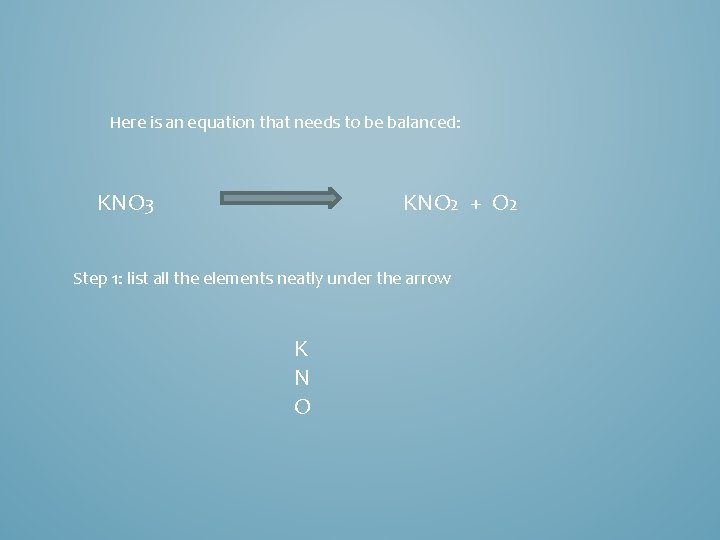Here is an equation that needs to be balanced: KNO 3 KNO 2 + O 2 Step 1: list all the elements neatly under the arrow K N OHere is an equation that needs to be balanced: KNO 3 KNO 2 + O 2 K N O Now add up how many K N and O you have on each sideHere is an equation that needs to be balanced: KNO 3 KNO 2 + O 2 1 K N O Now add up how many K N and O you have on each side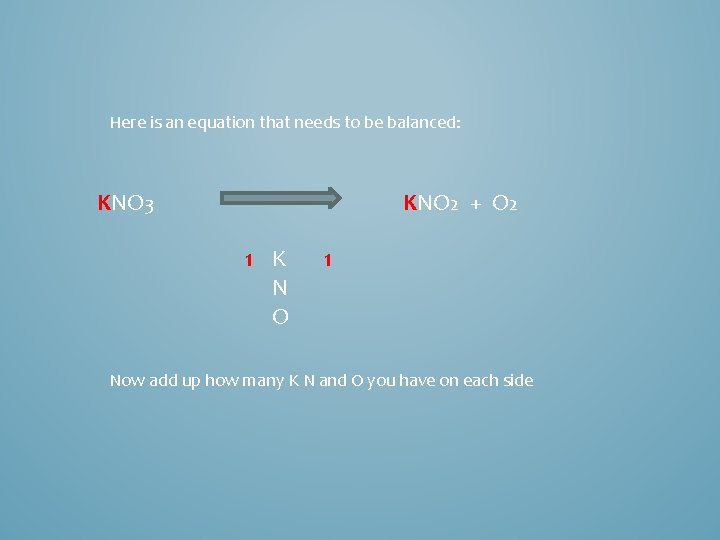Here is an equation that needs to be balanced: KNO 3 KNO 2 + O 2 1 K N O 1 Now add up how many K N and O you have on each side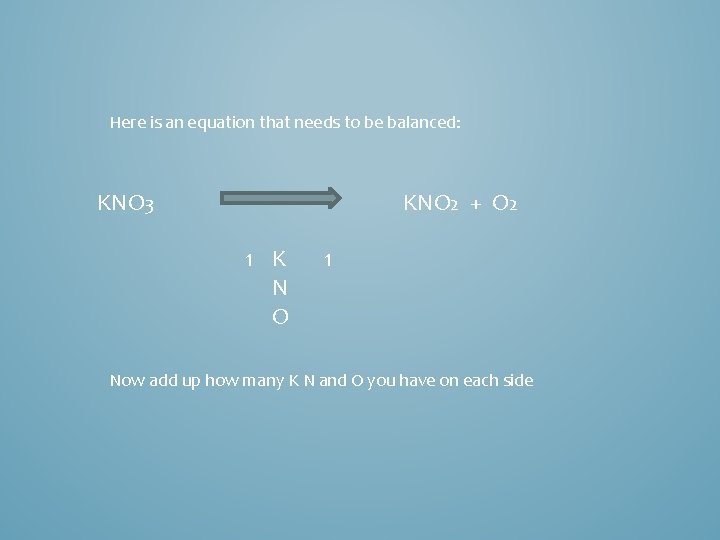Here is an equation that needs to be balanced: KNO 3 KNO 2 + O 2 1 K N O 1 Now add up how many K N and O you have on each side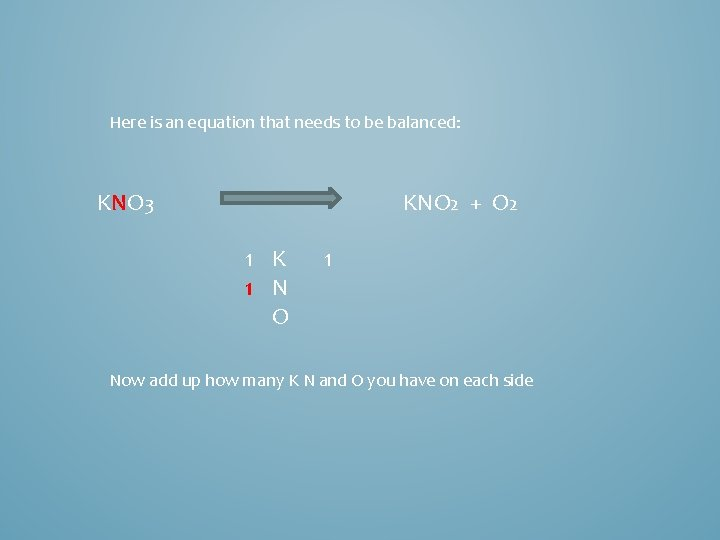Here is an equation that needs to be balanced: KNO 3 KNO 2 + O 2 1 K 1 N O 1 Now add up how many K N and O you have on each side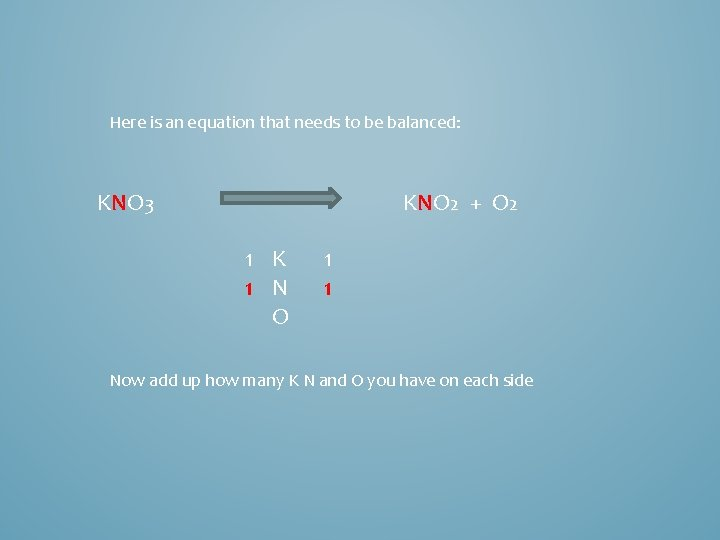Here is an equation that needs to be balanced: KNO 3 KNO 2 + O 2 1 K 1 N O 1 1 Now add up how many K N and O you have on each sideHere is an equation that needs to be balanced: KNO 3 KNO 2 + O 2 1 K 1 N O 1 1 Now add up how many K N and O you have on each sideHere is an equation that needs to be balanced: KNO 3 KNO 2 + O 2 1 K 1 N 3 O 1 1 Now add up how many K N and O you have on each side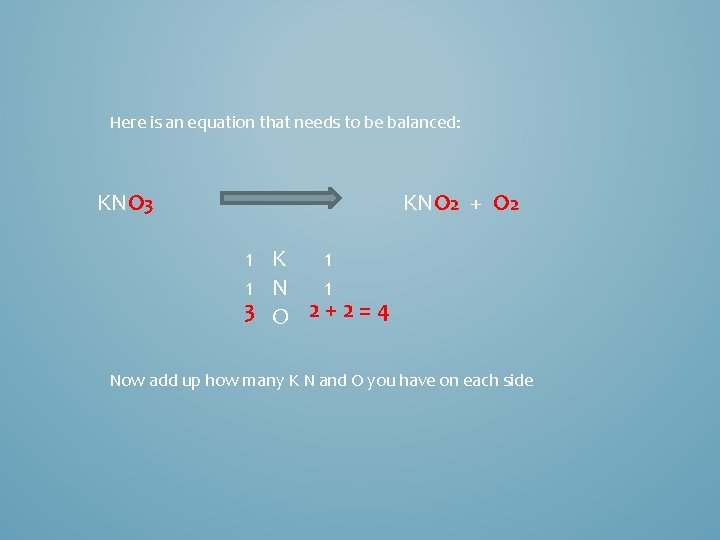Here is an equation that needs to be balanced: KNO 3 KNO 2 + O 2 1 1 K 1 N 1 3 O 2+2=4 Now add up how many K N and O you have on each side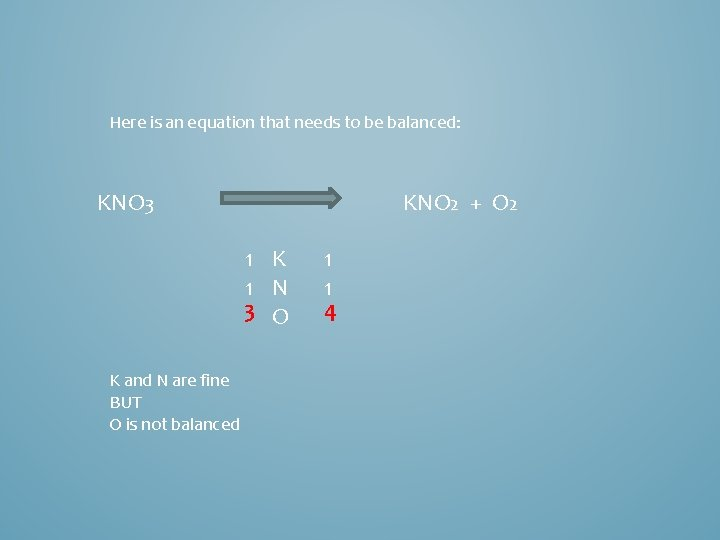Here is an equation that needs to be balanced: KNO 3 KNO 2 + O 2 1 K 1 N 3 O K and N are fine BUT O is not balanced 1 1 4Here is an equation that needs to be balanced: KNO 3 KNO 2 + O 2 1 K 1 N 3 O 1 1 4 We need more O on the left hand side We only want 1 more O BUT we can only have 1 KNO 3, or 2 KNO 3 or 3 KNO 3Here is an equation that needs to be balanced: KNO 3 KNO 2 + O 2 1 K 1 N 3 O 1 1 4 Lets see what happens if we have 2 KNO 3 on the left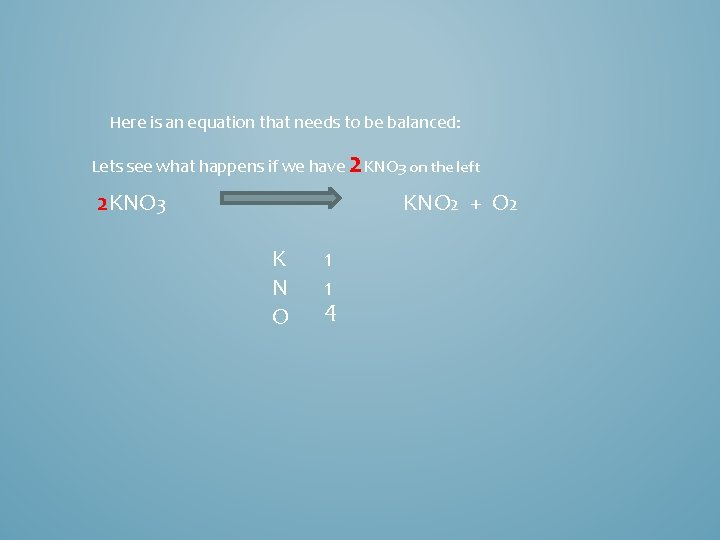Here is an equation that needs to be balanced: Lets see what happens if we have 2 KNO 3 on the left 2 KNO 3 KNO 2 + O 2 K N O 1 1 4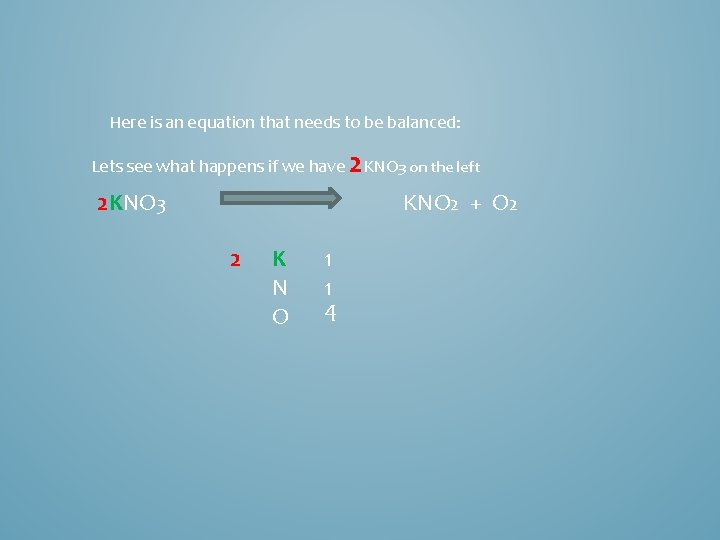Here is an equation that needs to be balanced: Lets see what happens if we have 2 KNO 3 on the left 2 KNO 3 KNO 2 + O 2 2 K N O 1 1 4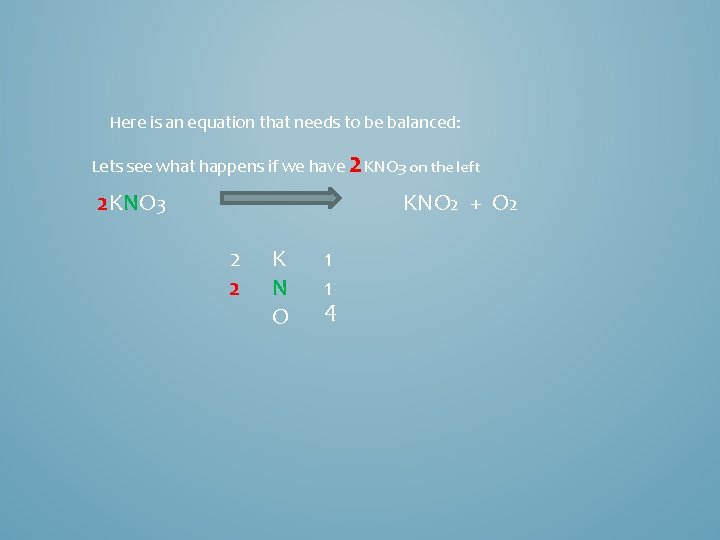Here is an equation that needs to be balanced: Lets see what happens if we have 2 KNO 3 on the left 2 KNO 3 KNO 2 + O 2 2 2 K N O 1 1 4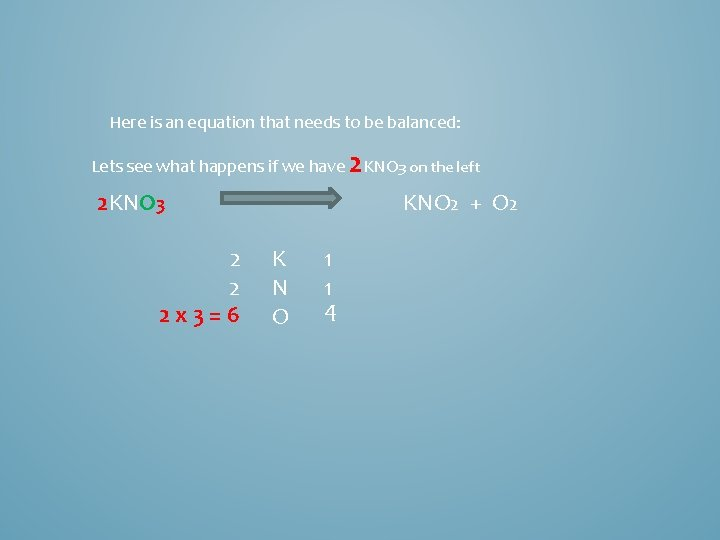Here is an equation that needs to be balanced: Lets see what happens if we have 2 KNO 3 on the left 2 KNO 3 2 2 2 x 3=6 KNO 2 + O 2 K N O 1 1 4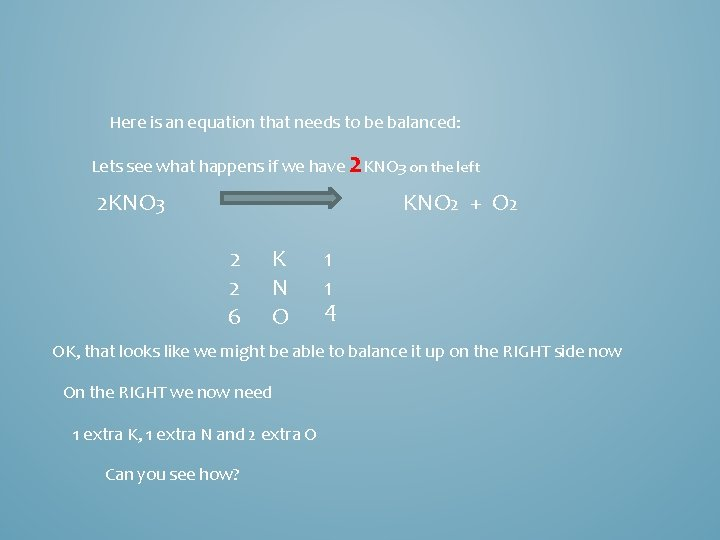Here is an equation that needs to be balanced: Lets see what happens if we have 2 KNO 3 on the left 2 KNO 3 KNO 2 + O 2 2 2 6 K N O 1 1 4 OK, that looks like we might be able to balance it up on the RIGHT side now On the RIGHT we now need 1 extra K, 1 extra N and 2 extra O Can you see how?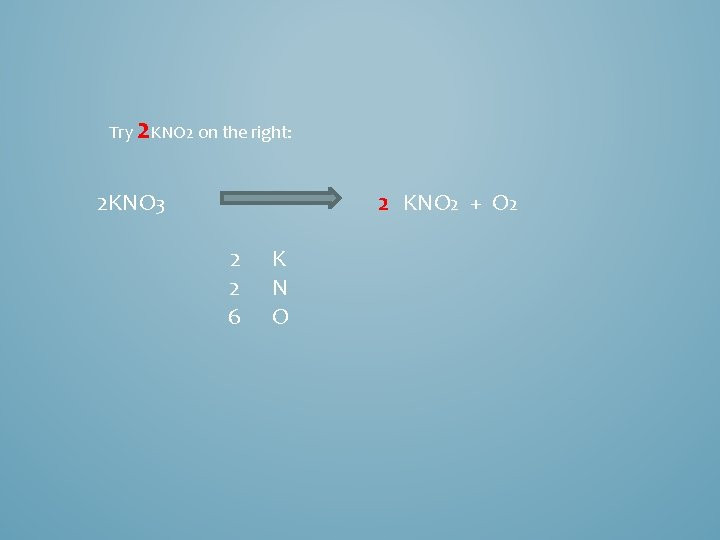Try 2 KNO 2 on the right: 2 KNO 3 2 KNO 2 + O 2 2 2 6 K N O2 KNO 3 2 KNO 2 + O 2 2 2 6 K N O 2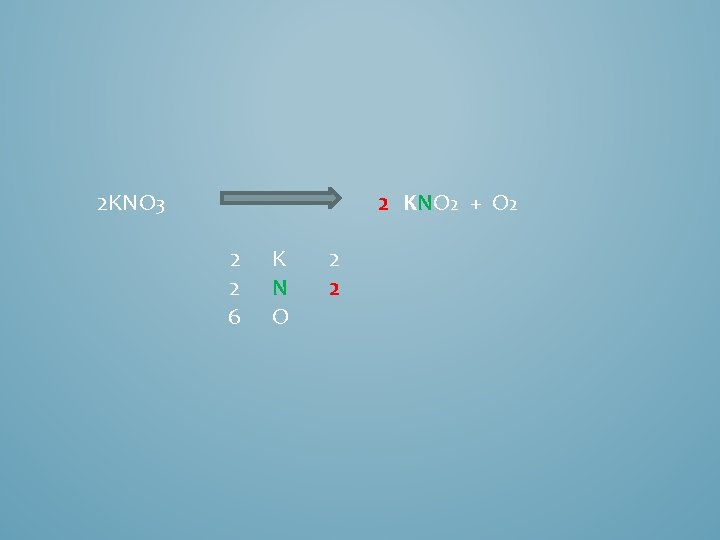2 KNO 3 2 KNO 2 + O 2 2 2 6 K N O 2 2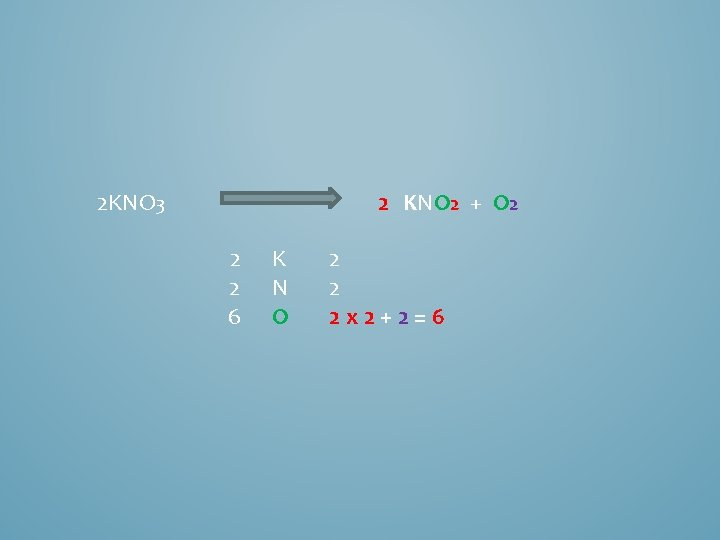2 KNO 3 2 KNO 2 + O 2 2 2 6 K N O 2 2 2 x 2+2=62 KNO 3 2 KNO 2 + O 2 2 2 6 K N O 2 2 6 That is balanced!!!!!